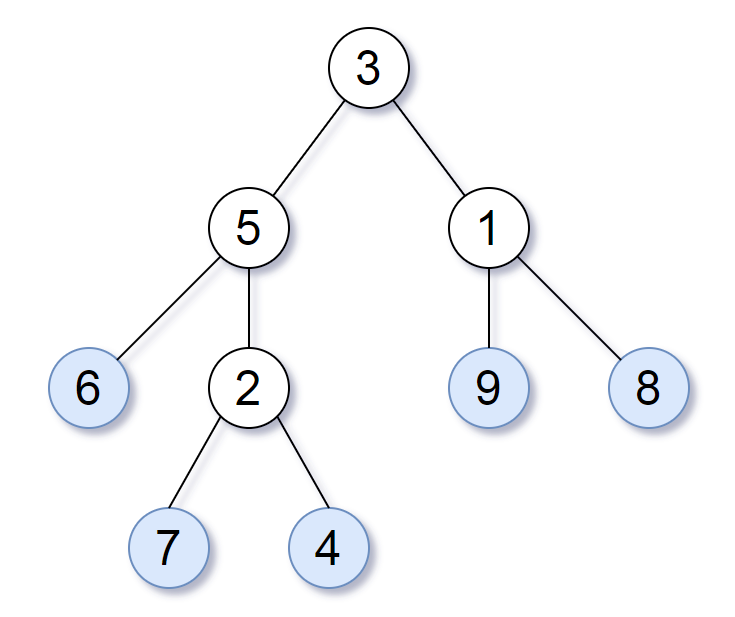### LeetCode - Tree - Leaf-Similar Trees

Consider all the leaves of a binary tree.  From left to right order, the values of those leaves form a leaf value sequence.For example, in the given tree above, the leaf value sequence is `(6, 7, 4, 9, 8)`.

Two binary trees are considered leaf-similar if their leaf value sequence is the same.

Return `true` if and only if the two given trees with head nodes `root1` and `root2` are leaf-similar.

```/**  * Definition for a binary tree node.  * public class TreeNode {  *     int val;  *     TreeNode left;  *     TreeNode right;  *     TreeNode(int x) { val = x; }  * }  */ class Solution {     List<Integer> r1_leafValues = new ArrayList<Integer>();     List<Integer> r2_leafValues = new ArrayList<Integer>();          public boolean leafSimilar(TreeNode root1, TreeNode root2) {         boolean result = true;                  getLeafValues(root1,r1_leafValues);         getLeafValues(root2,r2_leafValues);                  //System.out.println(r1_leafValues);         //System.out.println(r2_leafValues);                  if(r1_leafValues.size() != r2_leafValues.size()) result=false;         else{             for(int i=0;i<r1_leafValues.size();i++){                 if(r1_leafValues.get(i) != r2_leafValues.get(i)) result = false;             }         }         return result;     }          public void getLeafValues(TreeNode root, List leafValues){     if(root.left == null && root.right == null){         leafValues.add(root.val);     }     if(root.left !=null){         getLeafValues(root.left,leafValues);     }     if(root.right != null){         getLeafValues(root.right,leafValues);     }                 } }```